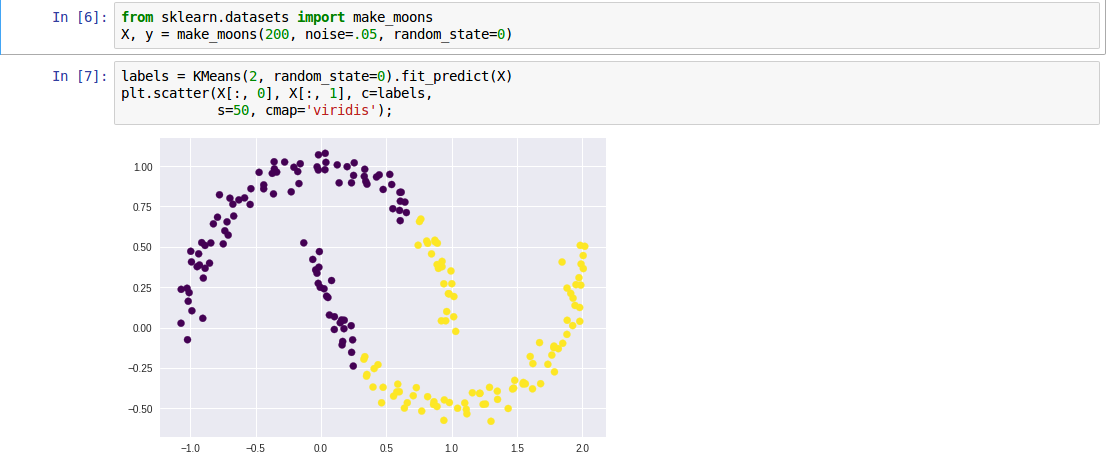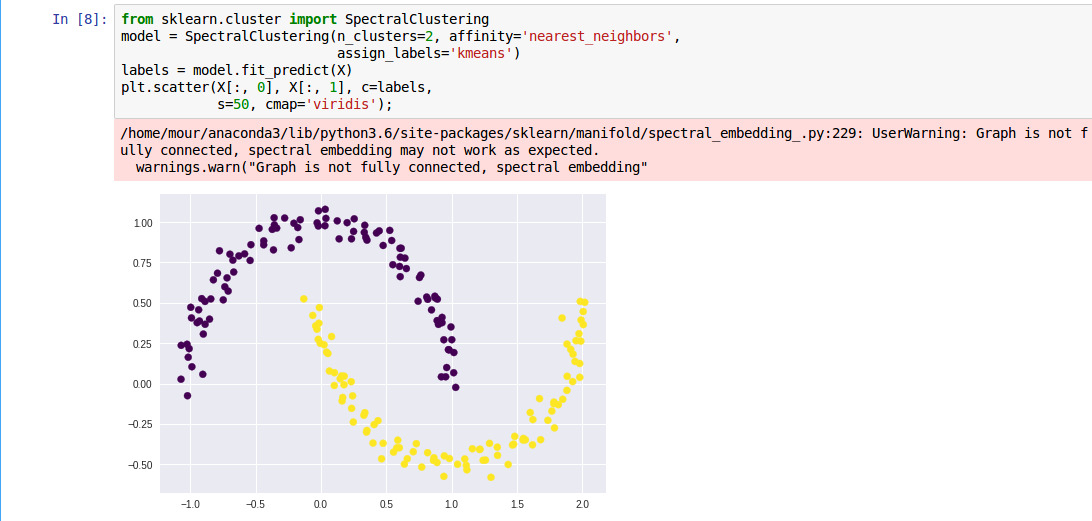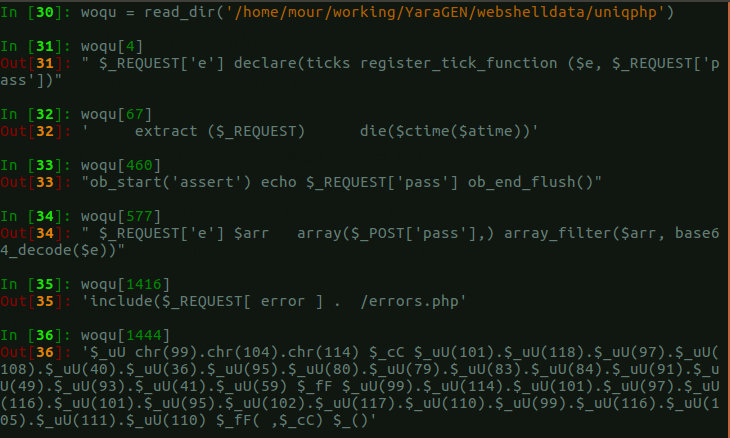# mylamour/blog

Have a question about this project? Sign up for a free GitHub account to open an issue and contact its maintainers and the community.

By clicking “Sign up for GitHub”, you agree to our terms of service and privacy statement. We’ll occasionally send you account related emails.

# Cluster Text Document With Spectral clustering #20

Open
opened this issue Apr 10, 2018 · 0 comments

Owner

### mylamour commented Apr 10, 2018```import os, sys, re
import logging
import pickle
import numpy as np
import matplotlib.pyplot as plt

from sklearn.datasets import make_moons
from sklearn.datasets.samples_generator import make_blobs

from sklearn.feature_extraction.text import HashingVectorizer, TfidfVectorizer
from sklearn.feature_extraction.text import TfidfTransformer
from sklearn.preprocessing import Normalizer

from sklearn.decomposition import TruncatedSVD
from sklearn.pipeline import make_pipeline
from sklearn.externals import joblib

from sklearn.cluster import SpectralClustering,KMeans

res = []
for r, d, f, in os.walk(folder):
for _ in f:
file = os.path.join(r, _)
res.append(" ".join(strings_file(file)))
return res

def strings_file(binfile):
chars = r"A-Za-z0-9/\-:.,_\$%'()[\]<> "
shortestReturnChar = 4
regExp = '[%s]{%d,}' % (chars, shortestReturnChar)
pattern = re.compile(regExp)
with open(binfile, 'rb') as f:

def strings2ascii(strings):
arr = [ord(c) for c in strings]
return arr, len(arr)

def clean_str(string):
"""
Tokenization/string cleaning for datasets.
Original taken from https://github.com/yoonkim/CNN_sentence/blob/master/process_data.py
"""
string = re.sub(r"[^A-Za-z0-9(),!?\'\`]", " ", string)
string = re.sub(r"\'s", " \'s", string)
string = re.sub(r"\'ve", " \'ve", string)
string = re.sub(r"n\'t", " n\'t", string)
string = re.sub(r"\'re", " \'re", string)
string = re.sub(r"\'d", " \'d", string)
string = re.sub(r"\'ll", " \'ll", string)
string = re.sub(r",", " , ", string)
string = re.sub(r"!", " ! ", string)
string = re.sub(r"\(", " \( ", string)
string = re.sub(r"\)", " \) ", string)
string = re.sub(r"\?", " \? ", string)
string = re.sub(r"\s{2,}", " ", string)
return string.strip().lower()

logging.basicConfig(level=logging.INFO, format='%(asctime)s %(levelname)s %(message)s')

n_features = 20000
n_components = 6

# Read dir file and single line in list

# Convert To Vectorizer Transform With TFIDF based on Hashing Vectorizer
hasher = HashingVectorizer(n_features=n_features, stop_words='english',
alternate_sign=False,
norm=None, binary=False)
vectorizer = make_pipeline(hasher, TfidfTransformer())

X = vectorizer.fit_transform(files)

# Dimensionality Reduction With LSA, also you can try with PCA
svd = TruncatedSVD(n_components)
normalizer = Normalizer(copy=False)
lsa = make_pipeline(svd, normalizer)
X = lsa.fit_transform(X)

# Use SpectralClustering Clustering
model = SpectralClustering(n_clusters=10, affinity='nearest_neighbors', assign_labels='kmeans')
model.fit(X)

labels = model.fit_predict(X)

# from sklearn import manifold
# X_embedded = manifold.TSNE(n_components=2).fit_transform(X)
# plt.scatter(X_embedded[:,0],X_embedded[:,1],labels)
# plt.show()

# import hypertools as hyp
# hyp.plot(X_embedded, '.', group=labels)

# Save
joblib.dump(model, 'spectral_clusering_webshell.pkl')
```
```for i,x in enumerate(labels):
if x == 1:
print(i)```# References

###mylamour added enhancement 工作 学习 安全 labels Apr 10, 2018

to join this conversation on GitHub. Already have an account? Sign in to comment
Projects
None yet
You can’t perform that action at this time.